GCSE Maths Algebra

Simultaneous Equations

# Simultaneous Equations

Here is everything you need to know about simultaneous equations for GCSE maths (Edexcel, AQA and OCR).

You’ll learn what simultaneous equations are and how to solve them algebraically. We will also discuss their relationship to graphs and how they can be solved graphically.

Look out for the simultaneous equations worksheets and exam questions at the end.

## What are simultaneous equations?

Simultaneous equations are two or more algebraic equations that share variables e.g. x and y.

They are called simultaneous equations because the equations are solved at the same time.

For example, below are some simultaneous equations:

2x + 4y = 14
4x − 4y = 4
6a + b = 18
4a + b = 14
3h + 2i = 8
2h + 5i = −2

Each of these equations on their own could have infinite possible solutions.

However when we have at least as many equations as variables we may be able to solve them using methods for solving simultaneous equations.

We can consider each equation as a function which, when displayed graphically, may intersect at a specific point. This point of intersection gives the solution to the simultaneous equations.

E.g.

\begin{aligned} x+y=6\\ -3x+y&=2 \end{aligned}

When we draw the graphs of these two equations, we can see that they intersect at (1,5).

So the solutions to the simultaneous equations in this instance are:

x = 1 and y = 5

### What are simultaneous equations?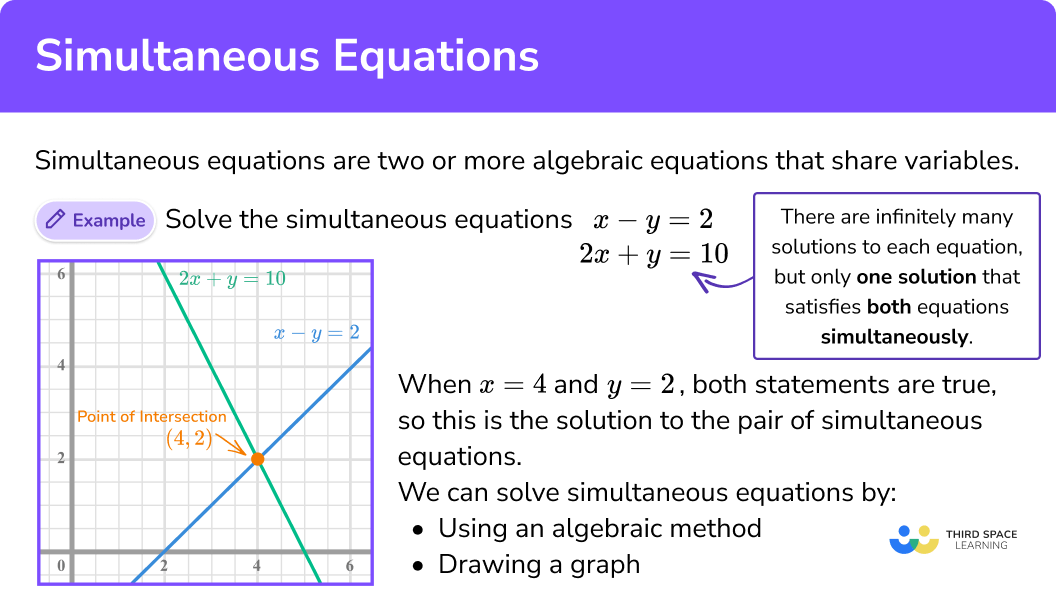## Solving simultaneous equations

When solving simultaneous equations you will need different methods depending on what sort of simultaneous equations you are dealing with.

There are two sorts of simultaneous equations you will need to solve:

• linear simultaneous equations

A linear equation contains terms that are raised to a power that is no higher than one.

E.g.

$2x + 5=0$

Linear simultaneous equations are usually solved by what’s called the elimination method (although the substitution method is also an option for you).

Solving simultaneous equations using the elimination method requires you to first eliminate one of the variables, next find the value of one variable, then find the value of the remaining variable via substitution. Examples of this method are given below.

A quadratic equation contains terms that are raised to a power that is no higher than two.

E.g.

$x^{2}-2x+1=0$

Quadratic simultaneous equations are solved by the substitution method.

### What are linear and quadratic simultaneous equations?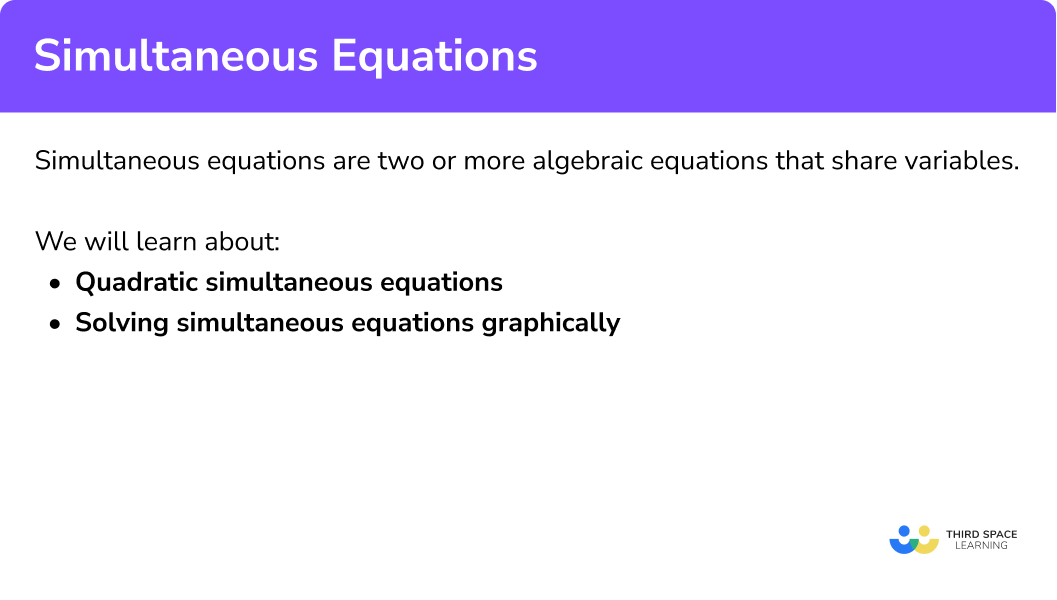## How to solve simultaneous equations

To solve pairs of simultaneous equations you need to:

1. Use the elimination method to get rid of one of the variables.
2. Find the value of one variable.
3. Find the value of the remaining variables using substitution.
4. Clearly state the final answer.
5. Check your answer by substituting both values into either of the original equations.

### How do you solve pairs of simultaneous equations?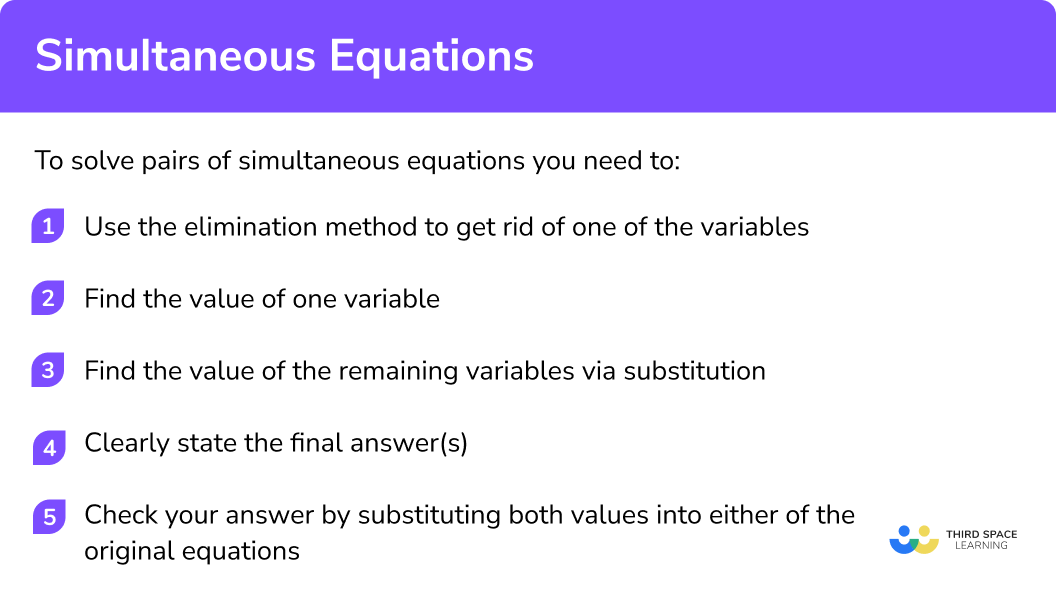See the examples below for how to solve the simultaneous linear equations using the three most common forms of simultaneous equations.

Quadratic simultaneous equations have two or more equations that share variables that are raised to powers up to 2 e.g. x^{2} and y^{2}.

Solving quadratic simultaneous equations algebraically by substitution is covered, with examples, in a separate lesson.

## Simultaneous equations examples

For each of the simultaneous equations examples below we have included a graphical representation.

Step-by-step guide: Solving simultaneous equations graphically

### Example 1: Solving simultaneous equations by elimination (addition)

Solve:

\begin{aligned} 2x+4y&=14\\ 4x-4y&=4 \end{aligned}

1. Eliminate one of the variables.

By adding the two equations together we can eliminate the variable y.

\begin{aligned} 2x+4y&=14\\ 4x-4y&=4\\ \hline 6x&=18 \end{aligned}

2Find the value of one variable.

3Find the value of the remaining variable via substitution.

We know x = 3 so we can substitute this value into either of our original equations.

$x=3 \qquad\qquad y=2$

5Check your answer by substituting both values into either of the original equations.

\begin{aligned} 4(3)+4(2)&=4\\ 12-8&=4\\ \end{aligned}

This is correct so we can be confident our answer is correct.

#### Graphical representation of solving by elimination (addition)

When we draw the graphs of these linear equations they produce two straight lines. These two lines intersect at (1,5). So the solution to the simultaneous equations is x = 3 and y = 2.

### Example 2: Solving simultaneous equations by elimination (subtraction)

Solve:

$\begin{array}{l} 6 a+b=18 \\ 4 a+b=14 \end{array}$

1. Eliminate one of the variables.

By subtracting the two equations we can eliminate the variable b.

\begin{aligned} 6a+b&=18 \\ 4a+b&=14 \\ \hline 2a&=4 \end{aligned}

NOTE: b − b = 0 so b is eliminated

2Find the value of one variable.

3Find the value of the remaining variable/s via substitution.

We know a = 2 so we can substitute this value into either of our original equations.

\begin{aligned} 6 a+b &=18 \\ 6(2)+b &=18 \\ 12+b &=18 \\ b &=6 \end{aligned}

$a=2 \qquad\qquad b=6$

5Check your answer by substituting both values into either of the original equations.

\begin{aligned} 4(2)+(6) &=14 \\ 8+6 &=14 \end{aligned}

This is correct so we can be confident our answer is correct.

#### Graphical representation of solving by elimination (subtraction)

When graphed these two equations intersect at (1,5). So the solution to the simultaneous equations is a = 2 and b = 6.

### Example 3: Solving simultaneous equations by elimination (different coefficients)

Solve:

$\begin{array}{l} 3 h+2 i=8 \\ 2 h+5 i=-2 \end{array}$

Notice that adding or subtracting the equations does not eliminate either variable (see below).

\begin{array}{l} 3 h+2 i=8 \\ 2 h+5 i=-2 \\ \hline 5 h+7 i=6 \end{array} \begin{aligned} 3 h+2 i&=8 \\ 2 h+5 i&=-2 \\ \hline h-3 i&=10 \end{aligned}

This is because neither of the coefficients of h or i are the same. If you look at the first two examples this was the case.

So our first step in eliminating one of the variables is to make either coefficients of h or i the same.

1. Eliminate one of the variables.

We are going to equate the variable of h.

Multiply every term in the first equation by 2.

Multiply every term in the second equation by 3.

\begin{aligned} 3h+2 i&=8 \\ 2h+5 i&=-2 \\ \\ 6h+4 i&=16 \\ 6h+15 i&=-6 \end{aligned}

Now the coefficients of h are the same in each of these new equations, we can proceed with our steps from the first two examples. In this example, we are going to subtract the equations.

\begin{aligned} 6 h+4 i&=16 \\ 6 h+15 i&=-6 \\ \hline -11 i&=22 \end{aligned}

Note: 6h − 6h = 0 so h is eliminated

Careful: 16 − − 6 = 22

2Find the value of one variable.

3Find the value of the remaining variable/s via substitution.

We know i = − 2 so we can substitute this value into either of our original equations.

$h=4 \qquad\qquad i=-2$

5Check your answer by substituting both values into either of the original equations.

\begin{aligned} 2(4)+5(-2)&=-2 \\ 8-10&=-2 \end{aligned}

This is correct so we can be confident our answer is correct.

#### Graphical representation of solving by elimination (different coefficients)

When graphed these two equations intersect at (1,5). So the solution to the simultaneous equations is h = 4 and i = − 2.

### Example 4: Worded simultaneous equation

David buys 10 apples and 6 bananas in a shop. They cost £5 in total.
In the same shop, Ellie buys 3 apples and 1 banana. She spends £1.30 in total.
Find the cost of one apple and one banana.

We need to convert this worded example into mathematical language. We can do this by representing apples with a and bananas with b.

\begin{aligned} 10a+6b&=5 \\ 3a+1b&=1.30 \end{aligned}

Notice we now have equations where we do not have equal coefficients (see example 3).

1. Eliminate one of the variables.

We are going to equate the variable of b.

Multiply every term in the first equation by 1.

Multiply every term in the second equation by 6.

\begin{aligned} 10 a+6 b&=5 \\ 3 a+1 b&=1.30 \\ \\ 10 a+6 b&=5 \\ 18 a+6 b&=7.80 \end{aligned}

Now the coefficients of b are the same in each equation we can proceed with our steps from the previous examples. In this example, we are going to subtract the equations.

\begin{aligned} 10a+6b &=5 \\ 18a+6b &=7.80 \\ \hline -8a &=-2.80 \end{aligned}

NOTE: 6b − 6b = 0 so b is eliminated

16 − − 6 = 22

2Find the value of one variable.

Note: we ÷ (− 8) not 8

3Find the value of the remaining variable/s via substitution.

We know a = 0.35 so we can substitute this value into either of our original equations.

$a=0.35 \qquad\qquad b=0.25$

So

1 apple costs £0.35 (or 35p) and 1 banana costs £0.25 (or 25p).

5Check your answer by substituting both values into either of the original equations.

\begin{aligned} 3(0.35)+1(0.25) & =1.30 \\ 1.05+0.25 & =1.30 \end{aligned}

This is correct so we can be confident our answer is correct.

#### Graphical representation of the worded simultaneous equatio

When graphed these two equations intersect at (1,5). So the solution to the simultaneous equations is a = 0.35 and b = 0.25.

### Common misconceptions

• Incorrectly eliminating a variable.
Using addition to eliminate one variable when you should subtract (and vice-versa).

• Errors with negative numbers.
Making small mistakes when +, −, ✕, ÷ with negative numbers can lead to an incorrect answer. Working out the calculation separately can help to minimise error.

Step by step guide: Negative numbers (coming soon)

• Not multiplying every term in the equation.
Mistakes when multiplying an equation. For example, forgetting to multiply every term by the same number.

• Not checking the answer using substitution.
Errors can quickly be spotted by substituting your solutions in the original first or second equations to check they work.

### Practice simultaneous equations questions

1. Solve the Simultaneous Equation

6x +3y = 48
6x +y =26Subtracting the second equation from the first equation leads to a single variable equation. Use this equation to determine the value of y , then substitute this value into either equation to determine the value of x .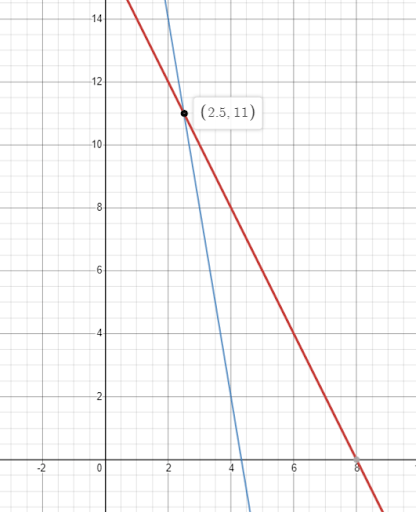2. Solve the Simultaneous Equation
x -2y = 8
x -3y =3Subtracting the second equation from the first equation leads to a single variable equation, which determines the value of y . Substitute this value into either equation to determine the value of x .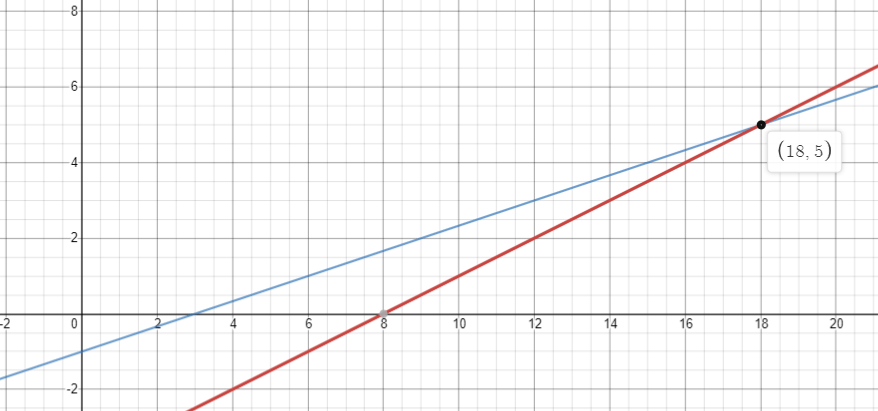3. Solve the Simultaneous Equation
4x +2y = 34
3x +y =21In this case, a good strategy is to multiply the second equation by 2 . We can then subtract the first equation from the second to leave an equation with a single variable. Once this value is determined, we can substitute it into either equation to find the value of the other variable.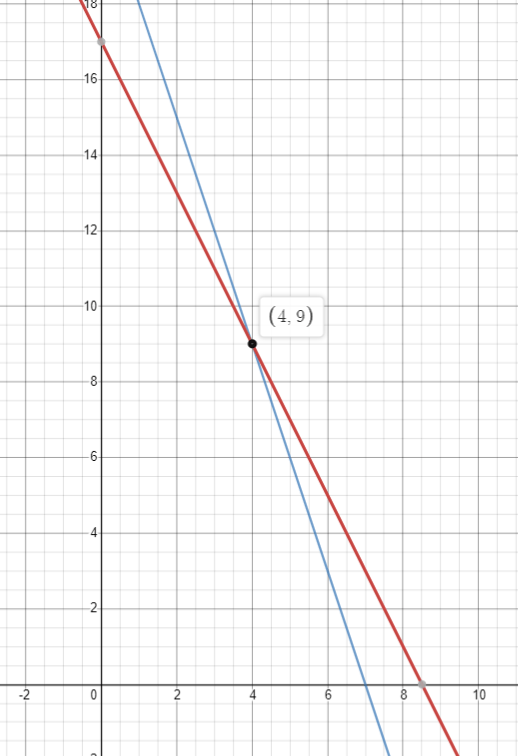4. Solve the Simultaneous Equation:

15x -4y = 82
5x -9y =12In this case, a good strategy is to multiply the second equation by 3 . We can then subtract the second equation from the first to leave an equation with a single variable. Once this value is determined, we can substitute it into either equation to find the value of the other variable.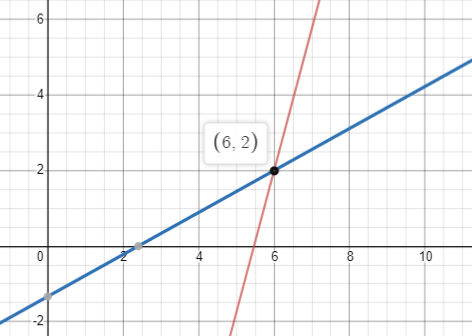### Simultaneous equations GCSE questions

1. Solve the simultaneous equations

\begin{array}{l} 3 y+x=-4 \\ 3 y-4 x=6 \end{array}

(4 marks)

\begin{array}{l} 5x=-10 \\ x=-2 \end{array}      or correct attempt to find y

(1)

One unknown substituted back into either equation

(1)

y=-\frac{2}{3} \text { oe }

(1)

x=-2

(1)

2. Solve the simultaneous equations

\begin{array}{l} x+3y=12 \\ 5x-y=4 \end{array}

(4 marks)

Correct attempt to multiple either equation to equate coefficients e.g.

\begin{array}{l} 5x+15y=60 \\ 5x-y=4 \end{array}

(1)

Or

\begin{array}{l} x+3y=12 \\ 15x-3y=12 \end{array}

(1)

Correct attempt to find y or x ( 16y=56 or 16x = 24 seen)

(1)

One unknown substituted back into either equation

ft (1)

y=\frac{7}{2} \text { oe }

x=\frac{3}{2} \text { oe }

(1)

3. Solve the simultaneous equations

\begin{array}{l} 4x+y=25 \\ x-3y=16 \end{array}

(4 marks)

Correct attempt to multiple either equation to equate coefficients e.g.

\begin{array}{l} 12x+3y=75 \\ x-3y=16 \end{array}

(1)

Or

\begin{array}{l} 4x+y=25 \\ 4x-12y=64 \end{array}

(1)

Correct attempt to find y or x ( 13x=91 or 13y=-39 seen)

(1)

One unknown substituted back into either equation

ft (1)

x=7 \text { oe }

y=-3 \text { oe }

(1)

## Learning checklist

• Solve two simultaneous equations with two variables (linear/linear) algebraically
• Derive two simultaneous equations, solve the equation(s) and interpret the solution

## Still stuck?

Prepare your KS4 students for maths GCSEs success with Third Space Learning. Weekly online one to one GCSE maths revision lessons delivered by expert maths tutors.

Find out more about our GCSE maths tuition programme.